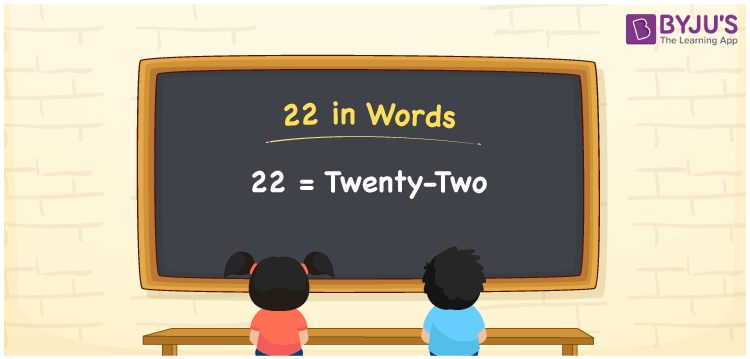# 22 in Words

22 in words is Twenty-Two. The number name of 20 can be written with the help of a place value chart. If you have 22 flowers in a garden, then you can say, “I have 22 flowers in my garden”. 22 is a cardinal number since it represents some specific amount.

 22 in words Twenty-Two Twenty-Two in numerical form 22

## 22 in English Words

Generally numbers in words are written using the English alphabet. Hence, we can read the number 22 in English as “Twenty-Two”.## How to Write 22 in Words?

Since 22 is a two-digit number. So, let us create a place value chart with two columns as given below

 Tens Ones 2 2

Here, ones = 2 and tens = 2

Now, let us expand these digits according to the place values

2 x Tens + 2 x Ones

= 2 x 10 + 2 x 1

= 20 + 2

= 22

= Twenty-Two

Therefore 22 in words is written as Twenty-Two

Interesting way of writing 22 in words

2 = Two

20 = Twenty

20 + 2 = 22

Twenty + two = Twenty-Two

Hence, the word form of 22 is Twenty-Two

22 is a natural number that is the successor of 21 and the predecessor of 23

• 22 in words – Twenty-Two
• Is 22 an odd number? – No
• Is 22 an even number? – Yes
• Is 22 a perfect square number? – No
• Is 22 a perfect cube number? – No
• Is 22 a prime number? – No
• Is 22 a composite number? – Yes

## Frequently Asked Questions on 22 in Words

### How do you write 22 in words?

22 in words can be written as Twenty-Two. Hence, in English 22 is expressed as Twenty-Two.

### How do you write Rs. 22 in words?

We can write Rs. 22 in words as Twenty-Two rupees.

### Write the value of 20 + 2 in words.

20 + 2 = 22 Twenty + two = Twenty-Two Hence, 20 + 2 is equal to Twenty-Two.